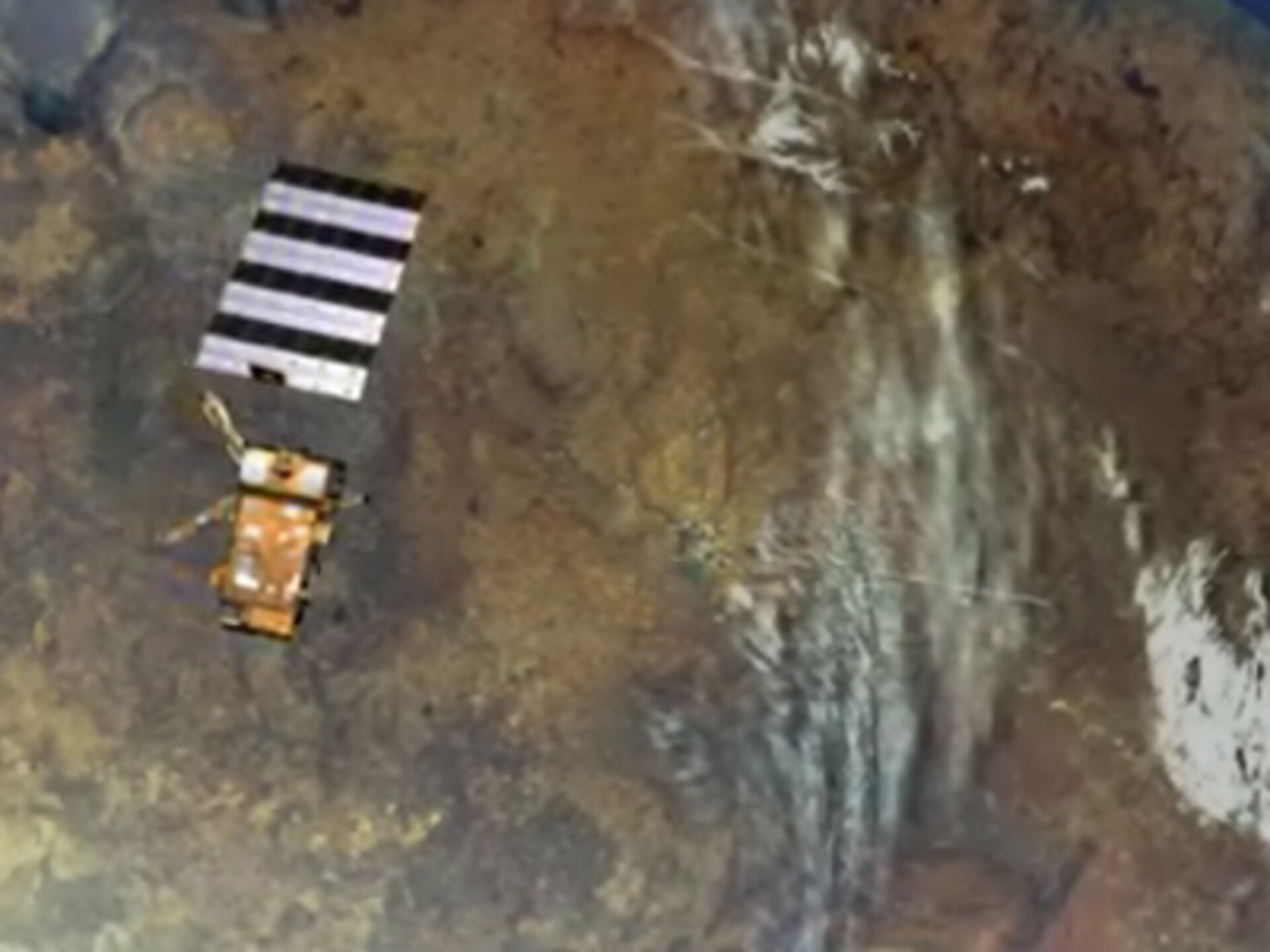Applications
403 views 0 likes

Flown on MetOp, the new GRAS (Global Navigation Satellite System Receiver for Atmospheric Sounding) instrument will, through the technique of radio occultation, provide very accurate information about the temperature and humidity in the atmosphere for use in weather forecasting and climate change monitoring.

Measurement principle
The nominal GPS constellation consists of 24 satellites distributed in six orbital planes around the globe. Each satellite orbit is circular with an inclination of 55°, a period of 12 h and an altitude of 20,200 km. A schematic of atmospheric profiling by GPS radio occultation is shown in the graphic.

An occultation occurs whenever a GPS satellite rises or sets on the Earth limbs as seen from the Low Earth orbit (LEO) satellite. The GPS signal is refracted and slowed as it traverses the Earth's atmospheric limb. This causes a phase delay that relates to characteristics of the Earth's atmosphere.

GRAS can track:
- up to eight satellites for navigation purposes
- two additional satellites for rise occultation measurements
- two others for set occultation measurements

For the receiver, an occultation occurs whenever a GPS satellite rises or sets and the ray path from its transmitter traverses the Earth's atmospheric limb. With 24 GPS satellites, a single GPS receiver in a near polar orbit at 824 km will observe over 500 occultations per day, distributed fairly uniformly about the globe.

Depending on the geometry of the orbits of the transmitter and receiver, occultations will have different shapes ranging from short to long duration.

The fundamental measurement in the GPS limb-sounding technique is the phase delay resulting from transmission of the GPS signal through the atmosphere. Total atmospheric delay is a function of two factors:

• ray bending due to refraction
• reduced propagation velocity in the atmosphere.

The radio signal propagating from the GPS transmitter to the low-Earth-orbiting receiver follows a path through the atmosphere that curves in response to atmospheric refractive-index gradients.

The cumulative effect of the atmosphere on the ray path can be expressed in terms of the total refractive bending angle (a) , which has a known relationship to the atmospheric Doppler shift. For the Earth's atmosphere, the maximum bending angle is in the order of 36 mrad (2°) at the Earth's surface, while at 30 km altitude its magnitude reduces to 0.3 mrad.

The atmospheric Doppler shift, in turn, is determined by taking the time derivative of the observed phase. The variation of a with experiment geometry can be characterised through the use of an impact parameter (a) defined as the perpendicular distance between the centre of the Earth and the straight line followed by the ray approaching the atmosphere. When combined with precise knowledge of the geometry (obtained concurrently from the navigation channels of the GPS receiver), each phase-data sample can be converted to the corresponding values for a and (a).

To extract information on the neutral atmosphere, propagation delays caused by the ionosphere must be isolated and removed from the signal. The correction required can be expressed as the difference in measured delay between the two GPS receiver channels at frequencies L1 and L2. The result is the bending angle for the neutral atmosphere an (a).

For an atmosphere with local spherical symmetry (i.e. no significant asymmetric horizontal variations in temperature or moisture) and having determined the bending angle an (a) as described above, there is a unique relationship between an (a) and m(r), the atmospheric refractive index as a function of radius r. The refractive index profile m(r) is then derived through an Abel transform of the measurements of an (a) obtained over a complete occultation.

For dry air at the top and above the troposphere, the refraction index can be expressed as a function of temperature and pressure as:
N(r) = [m(r) - 1] 106 = 77.6 P/T

Using the equation of state and integrating the equation of hydrostatic equilibrium, the temperature and pressure of the atmosphere for a given altitude can be determined.

Below the tropopause, the procedure described above needs to be modified to account for the presence of water vapour. The index of refraction can be expressed with two terms, the dry and wet terms. The second term, due to water vapour, exhibits considerable variations with location and time and increases considerably close to the Earth's surface.

The individual contributions to m(r) of the dry and wet terms cannot be distinguished uniquely through occultation measurements. Deeper in the troposphere, water-vapour concentrations increase, contributing to 30% of the total refractivity. A planned technique for recovery of the water vapour from measurements of m(r) is to use existing temperature analyses from meteorological offices. The accuracy to which lower tropospheric water-vapour profiles can be retrieved has been estimated by Kursinsky et al. at about 20%.